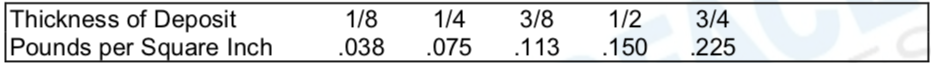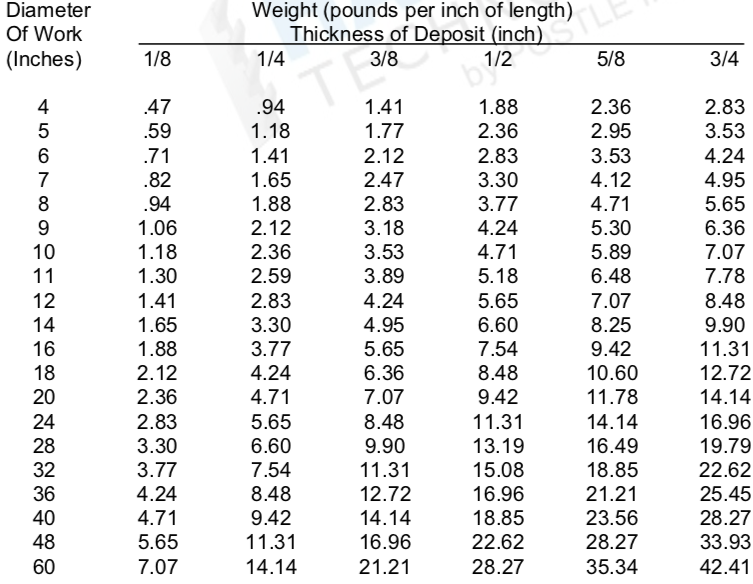# Determining How Much Hardfacing is Required

Squares and Rectangles: Length x Width x Depth x (.3) = Weight of Weld Deposit

Cylinders: 3.1416 x Diameter x Depth x (.3) = Weight of Weld deposit

To determine the amount of welding alloy required, divide the above answer by the deposit efficiency of the welding process.

Stick Electrode - 65%

Metal Core Wire - 95%

Bare TIG - 98%

Flux-Cored Wire - 90%

Table 1 - Weight of Weld deposit - Flat Work.

To estimate total weight of deposit, multiply weight per square inch by the number of square inches to be overlaid.Table 2 - Weight of Weld Deposit - Cylindrical Components.

To estimate total weight of deposit, multiply weight found below by the length (in inches) of the work to be overlaid.These figures are to be used only as an estimate. Find the diameter of cylindrical component in left-hand column and deposit depth in top row. Amount in proper column will give pounds of weld deposit per linear inch of the work. Multiply this amount by the length of work in inches. This number represents amount of weld metal deposited - factor in deposit efficiency of the process to estimate amount of welding alloy needed.